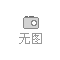• 全国 [切换]
• 二维码

扫一扫关注您所在的位置：直通车电子商务网`>`货源市集`>`家居网`>`书房家具`>`欧式家具 意大利家具 法式家具 古典家具 奢华家具 意大利手工雕花 全贴金箔 实木法官椅 法式奢华扶手椅1202扶手椅

## 佛山市南海沛俪菲帕家具厂

欧式家具,古典家具,实木家具,意大利风格家具,奢华家具,法式家具,手工雕花家具,意大利家居,别墅家具,巴洛克家具普通会员# 欧式家具 意大利家具 法式家具 古典家具 奢华家具 意大利手工雕花 全贴金箔 实木法官椅 法式奢华扶手椅1202扶手椅

产品价格面议

产品品牌未填

最小起订≥1 件

供货总量9 件

发货期限自买家付款之日起 天内发货

浏览次数613

更新日期2019-06-03 18:03

收藏商品 扫一扫 举报

## 诚信档案

### 佛山市南海沛俪菲帕家具厂

会员级别：企业会员

已  缴 纳：0.00 元保证金

我的勋章： [诚信档案]

企业二维码： 企业名称加二维码### 企业名片

佛山市南海沛俪菲帕家具厂

联 系  人：何思恩(先生)

电子邮箱：联系手机：联系固话：联系地址：广东省广州市琶洲保利世贸中心

【友情提示】：来电请说明在直通车电子商务网看到我们的，谢谢！

## 商品信息

基本参数

未填

广东

≥1 件

9 件

#### 有效期至：

长期有效
详细说明
rr r r r r r r r r r r r r r r r r r r r r r r r r r r r r r r
 r 包装体积:1.12r r 颜色分类:全贴金箔r r 风格:欧式r r 图案:艺术r r 附加功能:移动r r 家具结构:不规则体结构r r 产地:广东省r r 城市:佛山市r r 材质:木r r 木质材质:橡胶木r r 是否可定制:是r r 适用对象:成人r r 可送货/安装:佛山市r
rr rr

rr rr

rr rr

r rr

r rr

rr rr

rr rr

r r r r r r r r r r r r r r r r r r r r r r r r r r r r r r r r r r r r r r r r r r r r r r r r r r r r r r
 r 【名称】r r FP菲帕家居 古典家具 欧式手工雕花实木法官椅 法式奢华扶手椅r r 【风格】r r  欧式r r 【品牌】r r  菲帕家居（Filiphs Palladio）r r 【公司】r r  佛山市南海沛俪菲帕家具厂r r  【颜色】r r  全贴金箔r r 【同类推荐】r r  查看同系列配套产品 r r 【包装运输】r r  新型3CM厚蜂窝纸板包装r r 【产地】r r  广东佛山r r 【生产周期】r r  30-45天左右可发货 3-10天左右的运输期r r 【保养】r r  无须保养r r 【运费】r r  运费都是到付的 一般需要顾客到当地市区物流点自提货物r r 【产品尺寸】r r r 法官椅(长*宽*高):0.82*0.80*1.71m r r r 【材料】r r r  1.全实木框架(包括雕花部分)+胶合板+环保底漆+环保裂纹漆+台湾金箔+高密度海绵+优质布料,r 2.精选五金配件，经多次开合实验合格，回弹力度均匀;（传统风格的古典家具采用实木导轨，如喜欢推拉更顺畅的金属导轨，请与客服沟通）r r r 【包装箱数】r r    1件r r 【包装细节】r r  1.**层：产品;r 2.第二层：气泡膜+珍珠棉;r 3.第三层：3CM厚峰窝纸板搭建的板箱+护角纸r
rr r 法官椅(长*宽*高):0.82*0.80*1.71m r

rr rr

rr rr

rr rr

rr rr

r
0相关评论

换一批

## 相关栏目

还没找到您需要的书房家具产品？立即发布您的求购意向，让书房家具公司主动与您联系！

立即发布求购意向

#### 免责声明

本网页所展示的有关【欧式家具 意大利家具 法式家具 古典家具 奢华家具 意大利手工雕花 全贴金箔 实木法官椅 法式奢华扶手椅1202扶手椅_书房家具_佛山市南海沛俪菲帕家具厂】的信息/图片/参数等由直通车电子商务网的会员【佛山市南海沛俪菲帕家具厂】提供，由直通车电子商务网会员【佛山市南海沛俪菲帕家具厂】自行对信息/图片/参数等的真实性、准确性和合法性负责，本平台（本网站）仅提供展示服务，请谨慎交易，因交易而产生的法律关系及法律纠纷由您自行协商解决，本平台（本网站）对此不承担任何责任。您在本网页可以浏览【欧式家具 意大利家具 法式家具 古典家具 奢华家具 意大利手工雕花 全贴金箔 实木法官椅 法式奢华扶手椅1202扶手椅_书房家具_佛山市南海沛俪菲帕家具厂】有关的信息/图片/价格等及提供【欧式家具 意大利家具 法式家具 古典家具 奢华家具 意大利手工雕花 全贴金箔 实木法官椅 法式奢华扶手椅1202扶手椅_书房家具_佛山市南海沛俪菲帕家具厂】的商家公司简介、联系方式等信息。

#### 联系方式

在您的合法权益受到侵害时，欢迎您向邮箱发送邮件，或者进入《网站意见反馈》了解投诉处理流程，我们将竭诚为您服务，感谢您对直通车电子商务网的关注与支持！

### 按排行字母分类：

A B C D E F G H I J K L M N O P Q R S T U V W X Y Z

(c)2008-2018 DESTOON B2B SYSTEM All Rights Reserved